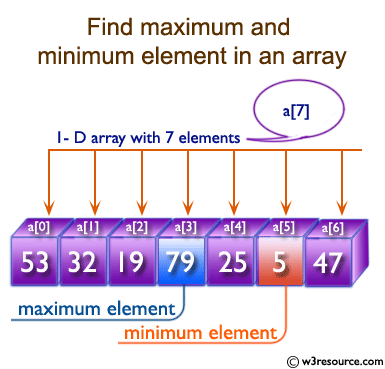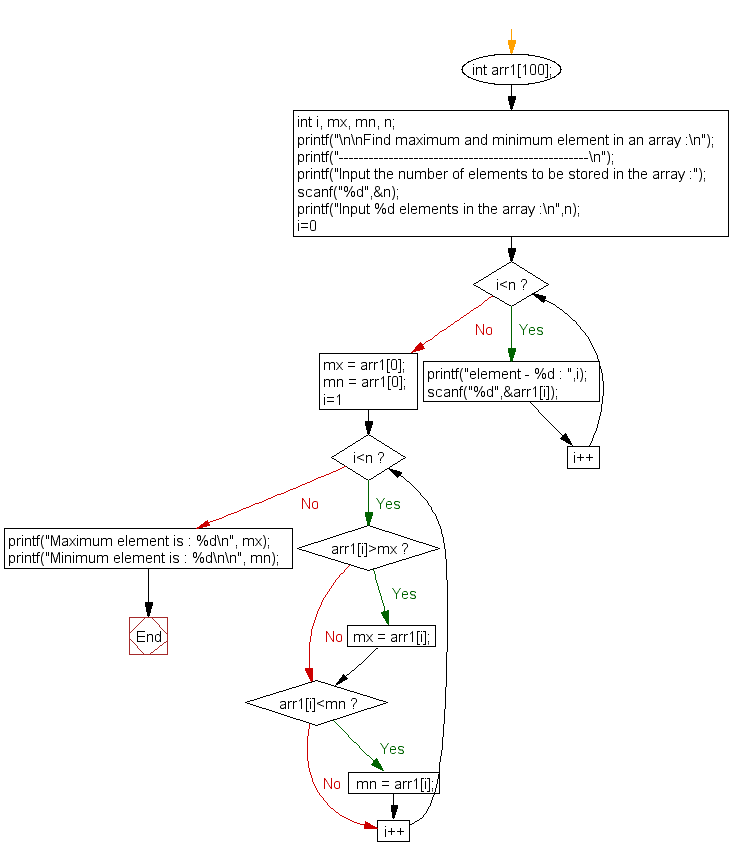﻿ C exercises: Find the maximum and minimum element in an array - w3resource# C Exercises: Find the maximum and minimum element in an array

## C Array: Exercise-9 with Solution

Write a program in C to find the maximum and minimum element in an array.

Pictorial Presentation:Sample Solution:

C Code:

``````#include <stdio.h>

void main()
{
int arr1;
int i, mx, mn, n;

printf("\n\nFind maximum and minimum element in an array :\n");
printf("--------------------------------------------------\n");

printf("Input the number of elements to be stored in the array :");
scanf("%d",&n);

printf("Input %d elements in the array :\n",n);
for(i=0;i<n;i++)
{
printf("element - %d : ",i);
scanf("%d",&arr1[i]);
}

mx = arr1;
mn = arr1;

for(i=1; i<n; i++)
{
if(arr1[i]>mx)
{
mx = arr1[i];
}

if(arr1[i]<mn)
{
mn = arr1[i];
}
}
printf("Maximum element is : %d\n", mx);
printf("Minimum element is : %d\n\n", mn);
}
```
```

Sample Output:

```Find maximum and minimum element in an array :
--------------------------------------------------
Input the number of elements to be stored in the array :3
Input 3 elements in the array :
element - 0 : 45
element - 1 : 25
element - 2 : 21
Maximum element is : 45
Minimum element is : 21
```

Flowchart:C Programming Code Editor:

Improve this sample solution and post your code through Disqus.

What is the difficulty level of this exercise?

﻿